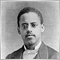# "wrong parameters count" with dependent classes18

Hi,

In an effort to simplify the problem I am having,  I have included two classe sin foo.mqh and bar.mqh

When I compile them, I get:

'bar' - wrong parameters count foo.mqh Line 20 Column 9

which is this line in foo.mqh:

foobar(bar  & b) { example = b;}

I have read up on other posts that deal with this error, but they don't seem to be object oriented and I can't  correllate those instances with this one.

Is it that bar has a default value? .... because of the constructor? Actually that is probably not it because if I put them in the same file I get the same error.

Is there anyway to get around this?

Any help would be appreciated. Thanks

```#property copyright "Copyright 2015, MetaQuotes Software Corp."
#property strict

class bar{
private:
int b;
int u;
int g;
public:
bar * operator=(const bar & example)
{
b = example.b;
u = example.u;
g = example.u;
return  GetPointer(this);
}
bar(bar & example)
{
b = example.b;
u = example.u;
g = example.u;
}

};```

```#property copyright "Copyright 2015, MetaQuotes Software Corp."
#property strict

#include<bar.mqh>

class foo {
};

class foobar: public foo {
private:
bar example;
public:
foobar(bar  & b) { example = b;}
bar getExample() { return example; }
};
```
Files:
bar.mqh 1 kb
foo.mqh 1 kb22605

In constructors you should get in the habit of using initialization lists, rather than creating sub-objects and then assigning to them.
 ```      bar(bar & example)       { // at this point b,u,g have already been constructed.          b = example.b;          u = example.u;          g = example.u;       }``` ```      bar(bar & example)        : b(example.b),          u(example.u),          g(example.u{}``` ```       foobar(bar  & b) {         // at this point bar example needs to be constructed.         // but all constructors require an argument.         // Thus misleading "wrong parameters count"         example = b;} ``` ```      foobar(bar  & b)            : example(b) { } ```